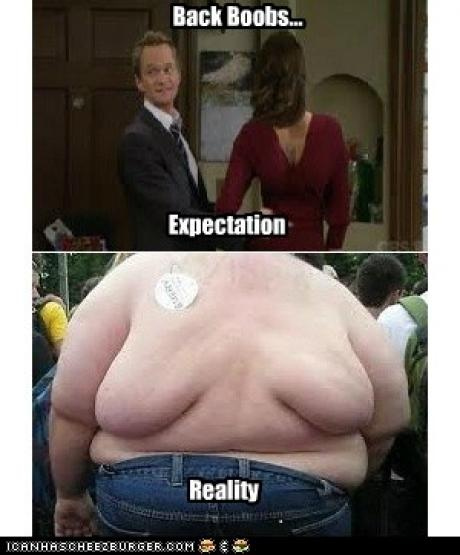Physics shit

These are the Physics definitions we need to know :) You're welcome ;)

Fleming's left hand ruleUsed with generators and electromagnetic induction. It shows the direction of the force on a conductor carrying a current in electric fields
Used with generators and electromagnetic induction. It shows the direction of the force on a conductor carrying a current in magnetic fields
Used with electric motors and forces acting on moving charges in magnetic fields. It shows the direction of the force on a conductor carrying a current in magnetic fields.
Used with generators and electromagnetic induction. It shows the direction of the force on a conductor carrying a current in electric fields.

StrangenessThe property of some quarks that is conserved in the strong
interaction, but not the weak interactions
The property of some quarks that is conserved in the weak
interactions, but not the strong interactions
A type of Quark
A particle containing 3 quarks

Magnetic flux densityA measure of the force acting upon an object in a magnetic field
A measure of the strength of the magnetic field
A measure of the distance the magnetic field acts upon
A measure of the force acting upon a charge in a magnetic field

Electric field strengthForce per unit negative charge
Positive charge per unit force
Force per unit positive charge
Negative charge per unit force

Fleming's right hand ruleUsed with electric motors and forces acting on moving charges in magnetic fields. It shows the direction of the force on a conductor carrying a current in magnetic fields.
Used with generators and electromagnetic induction. It shows the direction of the force on a conductor carrying a current in magnetic fields
Used with electric motors and forces acting on moving charges in electric fields. It shows the direction of the force on a conductor carrying a current in electric fields.
Used with generators and electromagnetic induction. It shows the direction of the force on a conductor carrying a current in electric fields